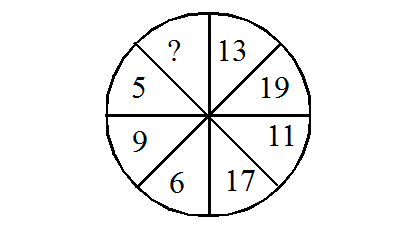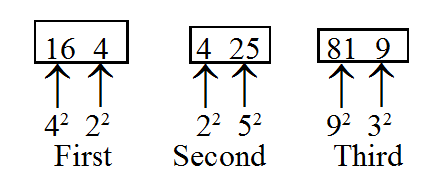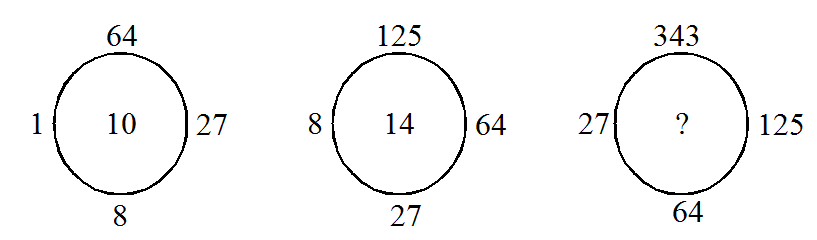# Number Puzzles - Questions with Answers for SSC Exam | Page-3

Questions

### Find the missing term in each of the following:

15A 25
B 36
C 49
D 64

Solution:

[(5 - 3) + (5 - 2)]2 = [2 + 3]2 = 25

Hence, missing term = [(7 - 2) + (4 - 3)]2 = [5 + 1]2 = 36

16A 10
B 9
C 8
D 7

Solution:

(6 × 2) + 1 = 13

(9 × 2) + 1 = 19

Hence, missing number = (8 × 2) + 1 = 17

17A 23
B 26
C 20
D 29

Solution:

7 × (7 - 1) = 42 ⇒ 42 + 3 = 45

4 × (4 - 1) = 12 ⇒ 12 + 3 = 15

6 × (6 - 1) = 30 ⇒ 30 + 3 = 33

8 × (8 - 1) = 56 ⇒ 56 + 3 = 59

3 × (3 - 1) = 6 ⇒ 6 + 3 = 9

Hence, missing number = 5 × (5 - 1) = 20 ⇒ 20 + 3 = 23

18A 130
B 142
C 128
D 138

Solution:

(111 + 25) - (43 + 35) = 136 - 78 = 58

So, the missing term = (48 + 180) - (34 + 56) = 228 - 90 = 138

19A 18
B 36
C 86
D 96

Solution:

We have (6 - 4)2 = 22 = 4

(8 - 5)2 = 32 = 9

(9 - 2)2 = 72 = 49

So, the missing number = (12 - 6)2 = 62 = 36

20A 819
B 791
C 899
D 550

Solution:21A 21
B 11
C 29
D 19